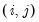maketransprobs
Save the regime transition probabilities and expected durations of switching VAR into the workfile.
Syntax
var_name.maketransprobs(options) [base_name]
var_name.maketransprobs(out=mat, options) [matrix_name]
where VAR_name is the name of a VAR estimated using switching regression.
In the first form of the command, base_name will be used to generate series names for the series that will hold the transition probabilities or durations. The series names for regime transition probabilities will be of the form base_name##, where ## are the indices representing elements of the transition matrix. The series names for expected durations will be of the form base_name# where # corresponds to the regime index. Thus, in a two-regime model, the base name “TEMP” corresponds to the transition probability series TEMP11, TEMP12, TEMP21, TEMP22, and the expected duration series TEMP1, TEMP2.
If base_name is not provided, EViews will use the default of “TPROB”
When the option “output=mat” is provided, the matrix_name is the name of the output matrix that will hold the transition probabilities or durations.
If matrix_name are not provided, EViews will default to “TPROB” or the next available name of the form “TPROB##”.
EViews will evaluate the transition probabilities or durations at the date specified by the “obs=” option. If no observation is specified, EViews will use the first date of the estimation sample to evaluate the transition probabilities. Note that if the transition probabilities are time-invariant, setting the observation will have no effect on the contents of the saved results.
Options

 type=arg (default=“trans”) Transition probability results to save: transition probabilities (“trans”), expected durations (“expect”). out=arg (default=“series”) Output format: series (“series”) or matrix (“mat”). If saved as a matrix, only a single transition matrix will be saved using the date specified by “obs=”. obs=arg Date/observation used to evaluate the transition probabilities if saving results as a matrix (“out=mat”). If no observation is specified, EViews will use the first date of the estimation sample to evaluate the transition probabilities.Note that if the transition probabilities are time-invariant, setting the observation will have no effect on the content of the saved results. n=arg (optional) Name of group to contain the saved transition probabilities. prompt Force the dialog to appear from within a program.
Examples
var var1.switchls(type=markov) y1 y2 y3
var1.maketransprobs(n=transgrp) trans
saves the transition probabilities in the workfile in the series TRANS11, TRANS12, TRANS21, TRANS22 and creates the group TRANSGRP containing the series.
The command
var1.maketransprobs(type=expect) AA
saves the expected durations in the series AA1 and AA2.
var1.maketransprobs(out=mat) BB
saves the transition probabilities in the matrix BB.
Cross-references
See “Switching VAR” for discussion.